telescopeѲptics.net          ▪▪▪▪                                             CONTENTS

8.4.4. Off-axis Newtonian

Off-axis Newtonian uses an off-axis mirror segment cut out of a larger paraboloid (parent mirror), so that it focuses outside the incoming pencil of light  (FIG. 136). The parent mirror has to be paraboloidal; off-axis segment from any other conic would suffer from spherical aberration, as well as from tilt induced center field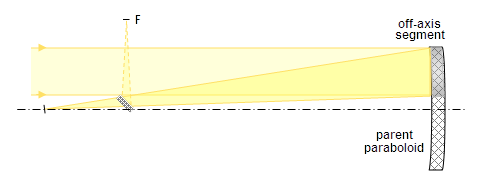FIGURE 136: Off-axis segment of a paraboloid focuses pencil of incoming light out of its path. Reflecting flat directing converging light to a more convenient location is also out of the incoming light path, allowing unobstructed system. The parent mirror is usually ~ƒ/4, which is close to the upper limit of its relative aperture, both, with respect to the economy of production and the amount of aberration leftover in the off-axis segment mirror (usually ƒ/10 to ƒ/12 effective ƒ-ratio). Segment of any other conic would produce axial aberrations.

coma and astigmatism, the farther from paraboloid, the more so (it is obvious for a sphere, whose any off-axis section is, in fact, a smaller sphere tilted with respect to the incoming light). The question is how much of the aberration of the parent mirror - and in what form - is passed onto its off-axis segment. The usual guess is that the main off-axis aberration of an off-axis section is also coma, only somewhat reduced. A glance at the aberrated wavefront of the parent mirror reveals that it is not so (FIG. 137, left). While the wavefront profile for the segment could be determined by  recalculating the coma pupil function for the segment area, it is easier and more illustrative to have it extracted from major points of the existing wavefront, assuming - with a degree of approximation - that the wavefront segment is purely astigmatic (that is, neglecting the low coma component).

With this assumption, the P-V error is determined by the peak aberration of the "parent" mirror wavefront along its axis of aberration, on one hand, and the segment size and position with respect to the wavefront on the other (FIG. 137, right). This is not a strict analysis - rather informal one and with approximate results, but illustrating the basis of transformation from the parent-mirror coma into off-axis segment astigmatism.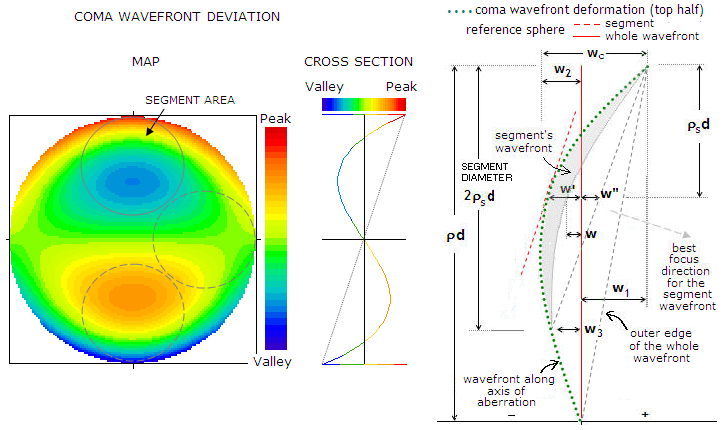FIGURE 137
: RIGHT: Along the axis of aberration (vertical axis), comatic wavefront deviation for a full aperture mirror has two lobe-like formations of opposite signs. Deformation of the portion of the wavefront reflected from an off-axis segment is mostly astigmatic, despite it being originating from mainly comatic deformation of the wavefront as a whole. Section of the wavefront reflected from the segment will have the radius along the axis of aberration more strongly curved than in the direction perpendicular to it. The P-V wavefront error of the segment is determined by the differential in these two perpendicular radii, over the wavefront area reflected from the segment. Since this astigmatic wavefront deformation originates from the parent mirror coma, it changes linearly, with the field angle - not with the square of it, as astigmatism does when it originates from the tilt of wavefront incident on surface of a rotationally symmetrical mirror (which is the "usual" astigmatism form for off-axis image points). Although segment can be cut out of some other portion of parent mirror (dashed circles), the astigmatic wavefront profile profile for off axis points does not change appreciably. The wavefront map suggests the opposite, at first: the side segment appears as having much less of wavefront deformation. However, the top and bottom segments don't have nearly as much of it as the map shows, since their best reference spheres are of different curvature than that for the entire wavefront, effectively reducing the deviation (as a result, segment focuses beams of different incident angles somewhat differently, which results in image tilt).
RIGHT: Wavefront profile along the axis of aberration (dotted green) for parent mirror's wavefront over its pupil radius d is the basis for calculating approximate P-V error of astigmatism (as the dominant aberration) in an off-axis section mirror. Perfect reference sphere for this wavefront (solid red) determines relative actual wavefront deviation at any pupil height ρ normalized to 1, according to ρ
3-(2ρ/3) (Eq. 12; for the axis of aberration, θ=0). For given segment diameter 2ρ'd, with its upper edge nearly coinciding with that of parent mirror's, the P-V wavefront error with respect to the same reference sphere can be approximated by w=w1+w2, with respect to the top and bottom point of the wavefront section. However, the section has an obvious tilt element in regard to this reference sphere, which is centered at the best focus for the comatic wavefront as a whole. Best focus of the wavefront segment lies in a slightly different direction, shifted downward with respect to the best focus direction of the parent mirror wavefront. Better reference sphere for the segment portion of the wavefront (dashed red) is the one with the same radius, but corrected for segment's tilt, with the P-V error along the axis of aberration - i.e. depth of wavefront radius in the vertical plane -  reduced to wSv~w'+w". However, since this section is of nearly spherical shape, most of the deviation does not represents wavefront error: it will nearly conform to a bit more strongly curved reference sphere. The actual P-V error results from the difference in curvatures along the vertical and horizontal cross sections of the segment's wavefront, the latter having radius depth wSh~w'-w.  Since the wavefront is more strongly curved vertically than in the direction perpendicular to it, its vertical section focuses closer than the horizontal section, with the radial sections focusing in between the tangential (formed by the vertical wavefront section) and sagittal focus. With the wavefront error being mainly astigmatic in its form, best focus lies near midway between the tangential and sagittal foci.

The above illustration shows that the relative P-V wavefront error of coma wc of this (top) half of the wavefront of parent mirror - in units of the peak aberration coefficient C in Eq. 12, with θ set to zero - with respect to the its reference sphere (solid red) over mirror radius d, is a sum of the absolute values of the two peak errors obtained from wc3-(2ρ/3) for ρ=1 (zonal height for the maximum positive deviation), denoted on above illustration as w1, and for ρ=0.47 (zonal height for the maximum negative deviation), denoted as w2. Thus, the sum of the two is w1+w2=0.33+0.21=0.54. It makes 81% of the relative P-V coma wavefront error wP (which is also in units of the peak aberration coefficient) over the entire parent mirror wavefront, given by a sum of wc3-(2ρ/3) for ρ=1 and ρ=-1, or 0.67, with respect to its reference sphere.

For practical reasons, all wavefront deviation values hereafter are given as absolute (i.e. positive).

However, with respect to the reference sphere adjusted for the tilt of the wavefront produced by the segment's surface, the relative P-V wavefront error at the center of the segment is somewhat smaller. In the horizontal plane, it is approximated - for practical relative (in units of parent mirror's radius d) segment radius ρS values of ~0.3 or greater - by wSh~w'+w", where w' is the relative (vs. parent mirror's) P-V wavefront error given by w'3-(2ρ/3) for radius value ρ=(1-ρS), and w"=(w1-w3)/2, with w3 obtained by substituting ρ=(1-2ρS), for ρ in ρ3-(2ρ/3).

The approximate sagitta value at the center of the segment along its vertical diameter is wSv~w'-w, with w being the wavefront deviation for the edge point on the segment's wavefront horizontal radius, with coordinates ρ and 1-ρ. The deviation is given by w=[ρ'3-(2ρ'/3)]cosθ, with ρ'=[ρ2+(1-ρ)2]1/2 and tanθ=ρ/(1-ρ).

In order to be expressed in units of the P-V wavefront error of the entire comatic wavefront of parent mirror wP, all wavefront deviation values are divided by 0.67. As already mentioned, ρS is the relative segment diameter in units of the parent mirror diameter.

The relative segment size ρS is limited by the number of segments cut out from the parent mirror. Usually, it is three or four which, for the theoretical limit given by ρSmax=1/[1+(1/sinβ)], with β=180/N in degrees, N being the number of equal-size segments, gives the maximum segment diameter ranging between 0.4 and 0.45 of the parent mirror diameter.

Taking ρS=0.4, centered at ρ=0.6, gives w' by substituting 1-ρS=0.6 for ρ in |ρ3-(2ρ/3)| as w'=0.184/0.67=0.28, in units of the P-V wavefront error of parent mirror. Likewise, w"=(w1-w3)/2, with w1=0.33 and w3=0.125, gives w"=0.1/0.67=0.15. Hence the tilt-adjusted P-V error for the segment's wavefront at its center in the horizontal plane as wSh~w'+w"=0.43wP, with wP being, as before, the coma P-V wavefront error of the parent mirror in units of the peak aberration coefficient C.

But this is not the effective P-V wavefront error for the segment. It is obvious that somewhat more strongly curved sphere is a better fit to this portion of the wavefront. Hence best focus for this wavefront section shifts somewhat closer (for light coming at the opposite angle, the section of reflected wavefront is weaker, with its best focus shifting somewhat farther; these foci shifts result in a tilted image surface, as explained in more detail a bit later).

The actual P-V error of the wavefront reflected from the segment results from its unequal cross-sectional radii. The P-V error equals the maximum sagitta differential, i.e. that between the vertical and horizontal wavefront radius, or wS=wSh-wSv. With the former approximated by wSh~0.48wc, and the latter obtained from wSv~w'-w, with w=[ρ'3-(2ρ'/3)]cosθ, with ρ'=[ρS2+(1-ρS)2]1/2 and tanθ=ρS/(1-ρS), hence w=0.09/0.67=0.13, and wSv~0.28-0.13=0.15, i.e. wSv~0.15wP, with the effective P-V error of the segment's wavefront as

wS=wSh-wSv=0.43wP-0.15wP~0.27wP

This is only slightly larger than the segment's wavefront error given by raytrace (wS~0.26wP). It implies that the P-V wavefront error of a typical off-axis segment is nearly four times smaller than the P-V coma wavefront error of the parent mirror at a given off-axis distance.

Applying the 0.26 factor to the coma P-V wavefront error expression for the parent mirror (Eq. 70), gives good approximation for the mainly astigmatic P-V wavefront error of such mirror segment as:

WS ~ αD/180F2     or, alternatively,      WS ~ h/180F3           (94)

with α being the field angle, h the linear height in the image plane, D the parent mirror aperture diameter and F the parent mirror focal ratio.

The raytrace gives, as expected, wavefront deformation form closely resembling that corresponding to best astigmatic focus (FIG. 138). Zernike coefficients below (OSLO, for 100mm ƒ/10 off-axis segment from 250mm ƒ/4 parent mirror) indicate that on-axis error is practically non-existent, as long as the mirror is perfectly paraboloidal (K=-1). Moderate figure error - in this case K=-0.95 conic with 250mm ƒ/4 parent mirror, resulting in 1/5.8 wave P-V of primary spherical aberration (best focus) - causes imperfect segment's wavefront as well. The dominant error component is astigmatism, with small amount of coma. The RMS error is 0.032 wave, or a bit over 60% of the on-axis RMS wavefront error of the parent mirror (which is, of course, spherical aberration). This RMS error carries over into the field, so at 0.13° off-axis the segment's wavefront error increases to 0.11 wave RMS.

 OFF-AXIS SEGMENT WAVEFRONT: ZERNIKE COEFFICIENTS ANALYSIS ZERNIKE ABERRATION ON AXIS 0.13° OFF-AXIS Z Name Polynomial (orthogonal) RMS const. K=-1 K=-0.95 K=-1 K=-0.95 Term value RMS Term value RMS* Term value RMS* Term value RMS* 0 Piston 1 - - - -0.00272 - 0.00008 - -0.00263 - 1 Tilt ρcosθ 1/2 - - - - -0.00009 0.00018 -0.00012 0.00024 2 ρsinθ - - - - - - - - 3 Defocus 2ρ2-1 1/√3 - - 0.00006 0.00003 0.00008 0.00005 0.00015 0.00009 4 Primary astigmatism ρ2cos2θ 1/√6 - - 0.07484 0.03055 0.18651 0.07614 0.26136 0.10670 5 ρ2sin2θ - - - - - - - - 6 Primary coma (3ρ3-2ρ)cosθ 1/√8 - - -0.03326 0.01176 -0.04022 0.01422 -0.07351 0.02599 7 (3ρ3-2ρ)sinθ - - - - - - - - 8 Primary spherical aberration 6ρ4-6ρ2+1 1/√5 - - 0.00278 0.00124 - - 0.00278 0.00124 9 Trefoil ρ3cos3θ 1/√8 - - -0.00007 0.00002 0.00019 0.00007 -0.00027 0.00010 10 ρ3sin3θ - - - - - - - - 11 Secondary astigmatism (4ρ4-3ρ2)cos2θ 1/√10 - - 0.00003 0.00001 0.00006 0.00002 0.00010 0.00003 12 (4ρ4-3ρ2)sin2θ - - - - - - - 13 Secondary coma (10ρ5-12ρ3+3ρ)cosθ 1/√12 - - -0.00002 0.00001 -0.00002 0.00001 -0.00003 0.00001 14 (10ρ5-12ρ3+3ρ)sinθ - - - - - - - - 15 Secondary spherical aberration 20ρ6-30ρ4+12ρ2-1 1/√7 - - - - - - - - Actual P-V/RMS wavefront error 0 / 0 0.17 / 0.033 0.38 / 0.078 0.55 / 0.11

*product of the RMS constant and Zernike coefficient's value

Other types of parent mirror surface errors will also affect correction level of the segment. Wide zonal deviation, for instance, will induce about as much of astigmatic P-V error to segment's mid field. Since it will be nearly offset by astigmatism induced due to entrance beam inclination, best focus would shift somewhat off field center. Local (or overall) roughness of parent mirror, however, would produce near identical roughness P-V - and possible proportionally larger roughness RMS - in the segment's wavefront.

Note that the RMS errors do not simply add arithmetically; the final wavefront shape results from the aggregate sum of positive and negative deviations at every wavefront point. Statistical error sum is given as the square root of the individual RMS wavefront errors squared. The RMS values indicate the magnitude of aberration contribution of the specific aberration forms to that final wavefront shape. When one aberration form strongly dominates, then its RMS error is a fairly good indicator of the RMS error of actual wavefront.FIGURE 138: Best focus wavefront deformation of an ƒ/10 off-axis section mirror (0.4D cut-out from 10" ƒ/4 parent mirror) at 0.16 degrees off-axis (perfect reference sphere is flat circle). The form of deformation is very similar to that of pure astigmatism, except that one of the tips of the "saddle" does not deviate as much relative to the center point as do the two bottoms (blue). In effect, one side of  the wavefront morphs toward cylindrical form, making this form of astigmatism sort of cross between best focus astigmatism on one, and sagittal or tangential astigmatism on the other side. This wavefront form results in slightly lower RMS-to-PV error ratio than that with "ordinary" astigmatism, as well as in triangular (instead of round) ray spot form at the best focus. The triangular form of pattern deformation probably makes this astigmatism form more similar to coma in appearance. It also "behaves" as coma, changing in proportion to the field radius, not its square, as the "regular" astigmatism does.

The RMS/PV ratio for pure astigmatism at the best focus is 1/24. For somewhat peculiar wavefront form produced by an off-axis segment of a paraboloid, OSLO gives larger RMS error, varying somewhat with the field point orientation, as shown on FIG. 140 (it also varies with ρ' value). The mean RMS/PV ratio here can be rounded off to ~1/23. Taking this RMS value, and applying it to the P-V wavefront error approximation (Eq. 94) gives the field RMS wavefront error (in units of the wavelength) of a typical paraboloidal off-axis segment as:

ω ~ h/860F3,          (94.1)

h being the linear height in the image plane in mm, and F the parent mirror F#. In units of 550nm wavelength, the RMS error is

ω' ~ 2.1h/F3,          (94.2).

Compared to the parent mirror's RMS wavefront error of coma (Eq. 70), quality linear and angular field size in the typical off-axis segment mirror is about 3.2 times larger, over its tilted best image surface. Since the quality linear field size changes in proportion to F3, that would make segment's linear field comparable to that in a paraboloid with the F# greater by nearly a factor of 1.5 than that of the parent mirror. In other words, with an ƒ/4 parent mirror, the segment with the above specs would have linear diffraction limited field comparable to an ƒ/6 paraboloid. And since quality angular field, for given aperture size, changes with F2, its quality angular/field is comparable to that in an equal-aperture (to parent mirror) paraboloid with F-ratio greater by nearly a factor of 1.8, or ƒ/7.2.

Setting ω=0.0745λ in Eq. 94.1 gives diffraction limited linear field radius of an off-axis segment as h~58λF3; in units of 550nm wavelength, it is:

hDL ~ fαDL ~ F3/28           (94.3)

with the  corresponding angular diffraction limited field

αDL ~ F2/28ρSD          (94.3.1)

with ρSD being the aperture diameter of the segment.

Comparison with Eq. 70.2 shows that its linear diffraction limited field is more than three times larger than in a paraboloid of identical aperture to that of the segment, and ƒ-ratio identical to the parent mirror. Assuming segment's ƒ-ratio larger by a factor of 2.5, this implies that segment's diffraction limited linear field is nearly 5 times smaller than in a paraboloid of identical ƒ-number and aperture.

Since the linear size of comatic blur changes with F3 only, and quality angular field with F2/D, an ƒ/4 paraboloid of the same aperture as the segment - in this case 2.5 times smaller than the parent mirror - has identical linear coma blur as the parent mirror - thus identical linear field - but since its focal length is 2.5 times smaller, it has as 2.5 times greater quality angular field. Since the quality angular (and linear) field of the segment is 3.2 larger than in the parent mirror, it implies that focal ratio of the equal-aperture paraboloid with comparable quality angular field is only slightly larger, by a factor of (3.2/2.5)0.5, or about 1.13F.

This can be confirmed by setting Eq. 94.3.1 equal to Eq.70.2.1 (with the focal ratio in the former being that of a paraboloid comparable at the segment's aperture level, and focal ratio in the latter being that of parent mirror) which, solved for the comparable focal ratio of a paraboloid equal in aperture to the segment, gives:

FA ~ 1.8Fρ0.5       (94.3.1)

where FA is the focal ratio of equal-aperture paraboloid with comparable field size to that in the segment, and F the parent mirror ƒ-ratio. For ρS~0.4, as before, that gives FA~1.13F.

With the mainly astigmatic blur in off-axis segment being nearly three times smaller than the comatic blur (tangential coma) at the same error level, typical segment has about 9 times smaller blur size at a given field height compared to its parent mirror.

The wavefront error diminishes with the relative segment size, nearly in proportion to S/0.4)2 or 6.25ρS2; for ρS=0.3, the RMS error is smaller by a factor ~0.56, thus ω~h/1500F3 or ω'~1.2h/F3 in units of 550nm wavelength, and the effective F# multiplying factors for its quality linear and angular field are nearly 1.8 and 2.4, respectively.

Since this wavefront error is, by its form, predominantly lower-order astigmatism of the sign opposite to that in most eyepieces, it is likely to be further reduced - and quality field expanded correspondingly - when used for visual observing. According to raytrace, 92mm ƒ/13 off-axis segment from 230mm ƒ/5.2 parent mirror, when used with 20mm symmetrical Plössl, has astigmatism rising to the diffraction-limited level (0.0745 wave RMS) at 0.135 degrees (2.8mm) off axis. The eyepiece alone has 0.1 wave RMS of astigmatism for the corresponding eyepiece field angle (~8°), which means that segment's astigmatism partly cancelling out that of the eyepiece resulted in about 15% wider linear diffraction limited field (it also shows that even at these slow focal ratios eyepiece astigmatism still dominates in the outer field).

Assuming this effect has similar magnitude with other conventional eyepieces, and at other eyepiece focal lengths (eyepiece astigmatism is proportional to its focal length, which means that the offset with the segment's astigmatism will be generally larger in focal lengths below 20 mm, and vice versa, so 20mm f.l. could represent a rough average), diffraction limited linear field of the segment in combination with these types of eyepieces would be better approximated by hDL~F3/24, with F, as in Eq. 94.3 being the parent mirror's focal ratio.

Another interesting property of an off-axis segment is its image tilt. Due to the wavefronts from different incident angles taking on different form of deviation after reflection from an off-axis segment - each form of deviation being a different portion of the original parent mirror comatic wavefront as a whole - they will not focus in the plane orthogonal to the line projected from segment's center to its center focus. Instead, they will form tilted astigmatic image surfaces, with best (median) image surface being at an angle to the straight line connecting  center of the segment and field center (FIG. 139 left). In the setup w/o flat, image on the same side of segment's central axis as the parent-mirror-edge-oriented segment radius tilts (rotates) away from the segment radius.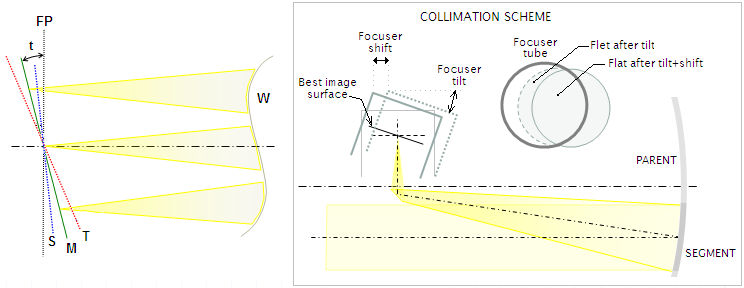FIGURE 139: LEFT: Image tilt formation in an off-axis section mirror. Curvatures of the reflected wavefronts vary with the angle of incidence, each wavefront being different section of the parent mirror's comatic wavefront (W). In the tangential (vertical) plane, the top image field point is formed by a less curved section of the parent wavefront, while the opposite image field point is formed by a more curved section. These wavefronts are also astigmatic, forming sagittal (S), tangential (T) and best, or median (M) field surface. The image tilt angle t is between the median image surface and focal plane (FP). Due to its origin in the comatic wavefront, this astigmatism changes linearly, and all three image surfaces are nearly flat. Its another odd property is that it diminishes to zero toward the perpendicular  (sagittal) field orientation, changing the sign on the opposite field side. Combined with the eyepiece astigmatism (which is of the same sign across the field), this gives best field definition in one direction, worst in the direction opposite to it, and intermediate in between (this would be occurring without any image tilt, but the two effects can combine). Image tilt, if not adjusted for, can significantly degrade off-axis performance of this type of mirror, with the exception of the image field portion near to the plane of tilt. RIGHT: Exaggerated collimation scheme of an off-axis segment mirror w/flat: adjusting focuser to the best image surface requires both tilting focuser toward primary by an angle nearly equal to the image tilt angle, and shifting it away from mirror to bring focuser axis back to the field center. This results in the flat moving from the center of the focuser tube toward mirror. Its final appearance in the focuser opening is determined by the tilt angle, flat-to-focuser separation and their respective dimensions. According to raytrace, between a 4-inch ƒ/10 and 6-inch ƒ/6.7, image tilt goes from 4.5º to 5.5º, respectively. Since no appreciable error results from up to ±1º deviation from the exact tilt angle - even somewhat larger - the needed tilt angle can be rounded off to about 5º for commercially available telescopes of this type. Needed focuser shift is given by h(tanτ), where h is the focus height above the bottom of the focuser base, and τ is the tilt angle.

For pure astigmatism, longitudinal aberration is given by LA=16WaF2 (note that Wa is half the P-V error, and F is the effective F#) which, for the above "average" segment, would approximate the image tilt angle υ as υ~23/F in degrees, with F being the parent mirror F#. The raytrace indicates it is somewhat smaller: υ~18/F or, for the segment mirror focal ratio F*, υ~46/F*, probably the consequence of somewhat different wavefront properties vs. that of pure astigmatism. The image tilt causes asymmetric image distortion in the plane orthogonal to the line connecting the field center with the center of the primary. The ray spot diagram on FIG. 140 shows image field of a 4" ƒ/10 off-axis segment cut out of a 10" ƒ/4 parent mirror.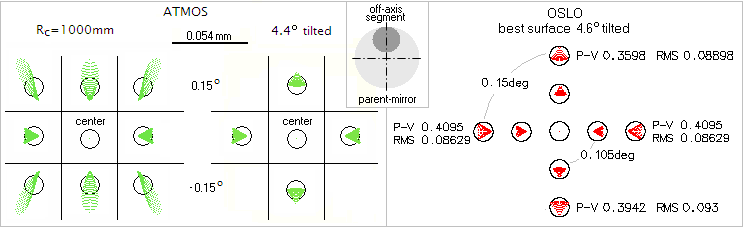FIGURE 140
: Ray spot diagram for a typical small off-axis Newtonian (4" ƒ/10), for 0.3º field diameter. To the left is its image field in ATMOS, over "regular" 1000mm-radius best image surface of parent mirror, The off-axis distortion comes from the image surface of the segment being tilted with respect to the Gaussian image plane. Next to it is a ray spot plot over the tilted (best) image surface, also in Atmos. Evidently, image tilt correction results in significant improvement in image field quality. The size of astigmatic blur is - as usual - deceiving: despite it being smaller than the Airy disc, the P-V wavefront error at 0.15º off-axis is about 0.5 wave (about 1/10 wave RMS, comparable to 1/3 wave P-V error of lower-order spherical aberration), for the 0.62 Strehl. With the error changing nearly in proportion to the off-axis height, it puts segment's diffraction-limited field radius at ~0.11°.
To the right is the same system in OSLO. It gives more detailed view of the same field, with PV/RMS errors along the two perpendicular field diameters. The wavefront error is somewhat smaller than that given by ATMOS, with diffraction limited field radius being over 0.12º (these values are used as the basis for Eq. 94/94.1, since ATMOS' output here may be compromised by it not adjusting the coma wavefront error for tilt correction; it triples the PV/RMS error, and results in much lower Strehl).
Below is somewhat wider field split vertically on ray and diffraction images (simulated by OSLO). Expectedly, diffraction images mainly show astigmatism (note that two vertical halves of the field are identical, while two horizontal halves are slightly different).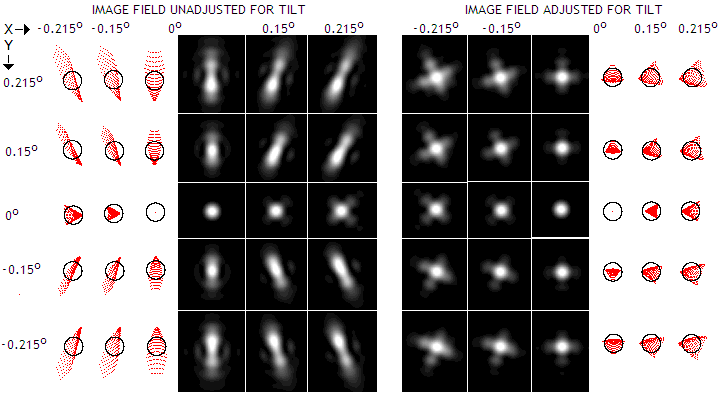Added significance of the off-axis paraboloid segment configuration is in it being relatively frequently employed with larger Newtonians using off-axis masks. The only difference is in the position of the aperture stop, which is in the "mask arrangement"  displaced from mirror surface to the mask. However, since the main aberration comes from the coma of the main mirror, and it is for a paraboloid independent of the stop position, the difference in the size of aberration between these two off-axis arrangements is negligible.